## Cipher## Mar 22, 2018

### Airline worker busted with 9 pounds of cocaine taped to legs - joke by the numbers

- Beginner level decode - 4 Base ciphers -

In 2017 there were a couple of  "smugglers" with cocaine taped to their legs, and I covered those all in one post , but we have another story, scripted by the numbers and coming exactly 1 year from one of the "smugglers" of 2017

CNN didn't provide us with much information but they gave us their sourceHugh Hall = 19+6+20+19 + 19+26+15+15 = 139 (Reverse Ordinal)

Cocaine = 24+12+24+26+18+13+22 = 139 (Reverse Ordinal)
nine pounds = 13+18+13+22 + 11+12+6+13+23+8 = 139 (Reverse Ordinal)

Montego Bay, Jamaica = 14+12+13+7+22+20+12 + 25+26+2 + 17+26+14+26+18+24+26 = 304 (Reverse Ordinal) - in numerology 0's are dropped, so 304 is seen as 34 (139 is the 34th prime number)

Jamaica to United States = 8+8+5+8+9+6+8 + 7+3 + 6+4+9+7+4+5 + 8+7+8+7+4+8 = 139(Reverse Reduction)

March 17th 2018 was a date with 31 numerology (3+17+2+0+1+8 = 31) - Hugh Hall = 1+6+2+1 + 1+8+6+6 = 31 (Reverse Reduction)

They refer to this guy as an "airline worker" in the headline

Airline worker = 1+9+9+3+9+5+5 + 5+6+9+2+5+9 = 77 (Full Reduction)
Hugh Hall = 8+21+7+8 + 8+1+12+12 = 77 (English Ordinal)
He was flying in from Montego Bay located at 77°W
There's always more ;)

## Feb 7, 2018

### SpaceX launches Tesla roadster into space

On February 6th we saw "Falcon heavy" launch with "Tesla roadster" and a dummy driver behind the wheel called "Starman"
They chose the perfect song for this trip

Starman = 8+7+8+9+5+8+4 = 49 (Reverse Reduction)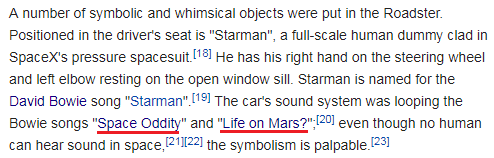CNN fails to mention that song "Life on Mars" is being played as well
Life on Mars = 3+9+6+5 + 6+5 + 4+1+9+1 = 49 (Full Reduction)
Mars is often referred to as the "Red Planet" = 49 (Reverse Full Reduction)

Also this was the 49th SpaceX rocket launch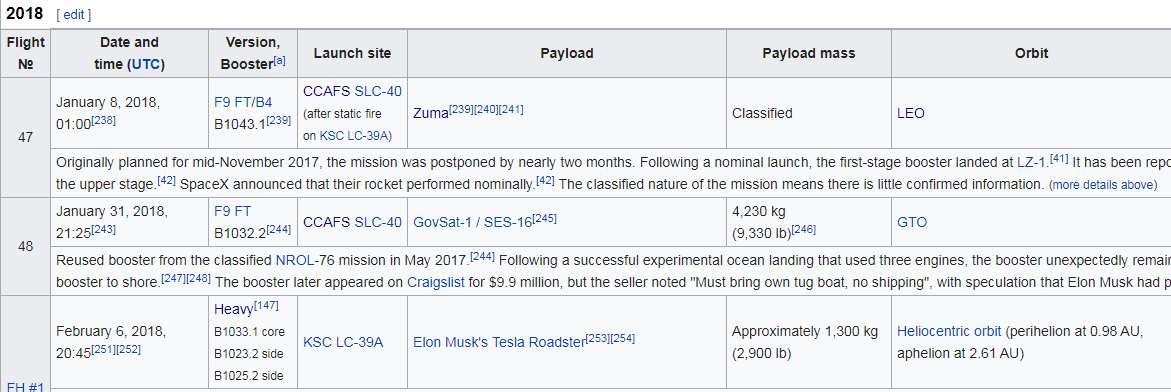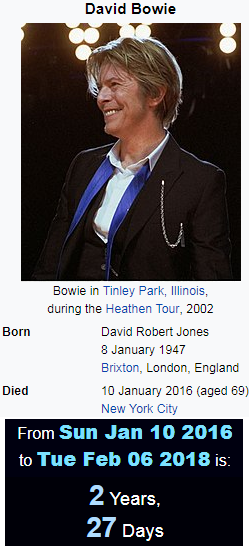Measuring from David Bowie's death to this rocket launch is 2 years and 27 days (49th prime number is 227)

SpaceX = 8+11+26+24+22+3 = 94 (Reverse Ordinal)
David Bowie = 4+1+22+9+4 + 2+15+23+9+5 = 94 (English Ordinal)

This level of mocking is unbelievable

## Jan 26, 2018

### September 11th - unbelievable predictive programming

Beginner level decode

In 1999 a synthpop duo called "I Am the World Trade Center" was founded and they released their first album "Out of the loop" a few months before 9/11/2001 with amazing predictive programming

A person not familiar with the practice of gematria would only see that the 11th track of this album is called "September", but with a piece of predictive programming like this you can except to find a lot more

This album was released on 7/17/2001 - prime factorization of 119 is 7x17
Name of the album also connects to 911 - Out of the loop = 12+6+7 + 12+21 + 7+19+22 + 15+12+12+11 = 156 (Reverse Ordinal)
I Am the World = 9 + 1+4 + 2+8+5 + 5+6+9+3+4 = 56 (Full Reduction)
From the release of this album to 9/11 attacks was a span of 56 days
There's always more

## Jan 21, 2018

### American Satan (movie) - 119 code - No spoilers

Beginner level decode (4 basic ciphers)
------------------------------------------------------------------------------------------------------------------------

This movie is basically about the music industry and making a deal with the devil in order to become famous and rich, the deal always seems to involve human sacrifice and it is full of occult symbolism.
American Satan = 1+13+5+18+9+3+1+14 + 19+1+20+1+14 = 119 (English Ordinal)
Initials also spell 119, A=1, S=19

The movie was released on 10/13/2017 - October thirteenth = 12+24+7+12+25+22+9 + 7+19+18+9+7+22+22+13+7+19 = 254 (Reverse Ordinal)
254th day of the year is 9/11
It came out on a date with 33 numerology (10+13+2+0+1+7), 33 days after 9/11/2017, September 11th is a day that leaves 111 days in the year and this movie is 111 minutes long, also this matches gematria of "October" = 12+24+7+12+25+22+9 = 111 (Reverse Ordinal)
October = 6+3+2+6+2+5+9 = 33 (Full Reduction)

In the movie the "Devil" calls himself Mr. Capricorn which also connects to 119

## Jan 19, 2018

### The Sun - random thoughts and 216

I was reading some Tesla quotes from this website and here's the quote that made me look again at the numbers surrounding the Sun

If you're into this kind of research you already know that Precession of the Equinoxes is a 25920 year cycle, which means that Sun spends 2160 years in each of the constellations (25920/12 = 2160)

216 = 6*6*6

In Kabbalah there's a hidden name of God containing 216 letters that is derived from 3 verses of Exodus Chapter 14 (verses 19, 20 and 21) , each of the verses contain 72 letters, and are arranged on top of each other, reading from top to bottom you find 72 hidden names of 3 letters.

72*3 = 216 (72 years is 1° of the Precessional cycle), Sun = 8+6+13 = 27 (Reverse Ordinal)

Think about the Magic Square of the Sun that adds up to 666 (36th triangular number),

Writing out the word "Sun" using reduction and reverse reduction ciphers shows interesting numbers

135+531 = 666

Now using reverse reduction we find 8-6-4 and one day contains 86,400 seconds
Also "Sun" has the value of 18 which can be written as 6+6+6

I find it very interesting how Sun has so many connections to 666
------------------------------------------------------------------------------------------------------------------------------------------------
Random bible verse

Here's a very interesting bible verse connecting 216 and 144, both numbers that in their own way connect to 666
They are talking about a wall of 144 cubits, which when measured in feet comes out to 216 as you see in the "New Living Translation"
New International Version has the best gematria though, check this out

Two one six = 20+23+15 + 15+14+5 + 19+9+24 = 144 (English Ordinal)

"and it was 144 cubits thick." = 1+14+4 + 9+20 + 23+1+19 + 3+21+2+9+20+19 + 20+8+9+3+11 = 216 (English Ordinal)

The Angel = 7+19+22 + 26+13+20+22+15 = 144 (Reverse Ordinal)

one hundred forty four = 6+5+5 + 8+3+5+4+9+5+4 + 6+6+9+2+7 + 6+6+3+9 = 108 (Full Reduction)

216 / 2 = 108

## Jan 9, 2018

### SpaceX launches Zuma satellite - Beginner Level

Beginner level decode

We have some textbook examples of Gematria usage in the mainstream media and scientific world

As you can see on this list, this was flight number 47

January 8th also happens to be a date with 47 numerology (1+8+20+18 = 47)

The satellite is named "Zuma" = 1+6+14+26 = 47 (Reverse Ordinal) Zuma satellite = 8+3+4+1 + 1+1+2+5+3+3+9+2+5 = 47 (Full Reduction)
Secretive satellite = 1+5+3+9+5+2+9+4+5 + 1+1+2+5+3+3+9+2+5 = 74 (Full Reduction)

Notice how CNN pays tribute to the manufacturers of this spacecraft in their headline gematriaSpaceX launches secretive Zuma spacecraft = 8+2+8+6+4+3 + 6+8+6+4+6+1+4+8 + 8+4+6+9+4+7+9+5+4 + 1+6+5+8 + 8+2+8+6+4+6+9+8+3+7 = 211 (Reverse Reduction)

I also find this interesting

Peace = 60+5+1+3+5 = 74 (Jewish)

## Oct 26, 2017

### Las Vegas shooter's brother charged with having child porn - Bruce Paddock

Short post on another ridiculous story

So Bruce Paddock has been charged with possession of more than 600 images of child porn and 19 counts of sexual exploitation of a child
nineteen counts of sexual exploitation of a child = 193 (Full Reduction)
44th prime is 193
pornography = 11+12+9+13+12+20+9+26+11+19+2 = 144 (Reverse Ordinal)
porn = 11+12+9+13 = 45 (Reverse Ordinal)
pornography = 2+3+9+4+3+2+9+8+2+1+2 = 45 (Reverse Reduction)
How fitting..
Las Vegas shooting took place the day after one of the most important holidays in the Jewish year, Yom Kippur ( Day of Atonement)
Bruce Paddock is currently 59 years old, and supposedly 59 people died on October 1st including his brother
Paddock = 16+1+4+4+15+3+11 = 54 (English Ordinal)
Fifty nine = 6+9+6+2+7 + 5+9+5+5 = 54 (Full Reduction)
Note how they end this with the least relevant information anyone could ask for - why do you need to know that he once worked about a 45 minute drive northwest of LA?

It's all a joke

## Aug 26, 2017

### Jay Thomas (actor) dies of cancer at 69

Jon Thomas Terrell = 17+12+13 + 7+19+12+14+26+8 + 7+22+9+9+22+15+15 = 227(Reverse Ordinal)
Jay is born in the sign of Cancer and he dies from cancer at 69 on a date with 69 numerology - 8+24+20+17 = 69... How can this be a coincidence?
He died 44 days after his birthday connecting to "Cancer" = 3+1+14+3+5+18 = 44 (English Ordinal)
44 days is 1056 hours - He died in Santa Barbara, California = 8+8+4+7+8 + 7+8+9+7+8+9+8 + 6+8+6+9+3+3+9+4+9+8 = 156 (Reverse Full Reduction)
156th prime number is 911
He also died 10 months and 19 days before his next birthday including the end date
Cancer = 24+26+13+24+22+9 = 118 (Reverse Ordinal)
Death = 4+5+1+100+8 = 118 (Jewish)

His last appearance was on a TV show 'Ray Donovan' and the episode aired on 8/25/2013 exactly 4 years before we see the news of his death in the media

There's more to find but this one is very obvious

## Aug 18, 2017

### Deadly van attack in Spain, vehicle ramming attacks are trending

http://www.cnn.com/2017/08/17/europe/barcelona-spain-van-latest/index.html
This has become a monthly thing now...DEADLY VAN ATTACK IN SPAIN = 4+5+1+4+12+25 + 22+1+14 + 1+20+20+1+3+11 + 9+14 + 19+16+1+9+14 = 226 (English Ordinal)
August Seventeenth = 1+21+7+21+19+20 + 19+5+22+5+14+20+5+5+14+20+8 = 226(English Ordinal)
thirteen killed = 7+19+18+9+7+22+22+13 + 16+18+15+15+22+23 = 226 (Reverse Ordinal)
ISIS terror attack = 18+8+18+8 + 7+22+9+9+12+9 + 26+7+7+26+24+16 = 226(Reverse Ordinal)41 is the 13th prime number
Barcelona, Spain = 2+1+18+3+5+12+15+14+1 + 19+16+1+9+14 = 130 (English Ordinal)
This attack is happening in the sign of  "Leo" = 6+4+3 = 13 (Reverse Reduction)
"Deadly van attack" = 4+5+1+4+3+7 + 4+1+5 + 1+2+2+1+3+2 = 45 (Full Reduction)
Thirteen = 2+8+9+9+2+5+5+5 = 45 (Full Reduction)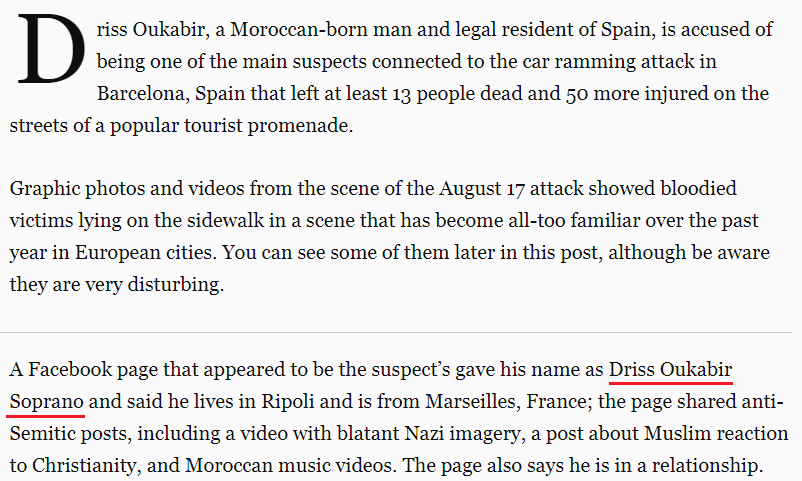Suspect - Driss Oukabir Soprano = 4+9+9+1+1 + 6+3+2+1+2+9+9 + 1+6+7+9+1+5+6 = 91 (Full Reduction) - the 13th triangular number is 91
August Seventeenth = 26+6+20+6+8+7 + 8+22+5+22+13+7+22+22+13+7+19 = 233(Reverse Ordinal) - 233 is the 13th Fibonacci number and we see "At least thirteen dead" = 26+7 + 15+22+26+8+7 + 7+19+18+9+7+22+22+13 + 23+22+26+23 = 322 (Reverse Ordinal)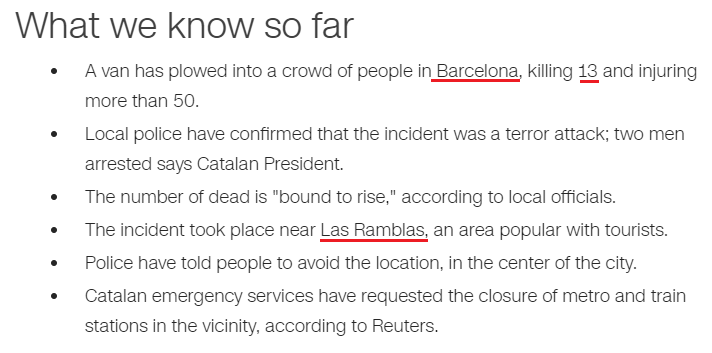"The incident took place near "Las Ramblas" = 15+26+8 + 9+26+14+25+15+26+8 = 172 (Reverse Ordinal)
Barcelona = 25+26+9+24+22+15+12+13+26 = 172 (Reverse Ordinal)Islamic State of Iraq and Syria = 9+1+3+1+4+9+3 + 1+2+1+2+5 + 6+6 + 9+9+1+8 + 1+5+4 + 1+7+9+9+1 = 117 (Full Reduction)
Central Intelligence Agency = 3+5+5+2+9+1+3 + 9+5+2+5+3+3+9+7+5+5+3+5 + 1+7+5+5+3+7 = 117 (Full Reduction)
CIA = 3+9+1 = 13 (English Ordinal)
Thirteen = 7+19+18+9+7+22+22+13 = 117 (Reverse Ordinal)Mike Pence = 4+9+2+5 + 7+5+5+3+5 = 45 (Full Reduction)
Thirteen = 2+8+9+9+2+5+5+5 = 45 (Full Reduction)
Mike Pence is giving a speech on 229th day of the year, exactly 9 months and 22 days before his next birthdayNote that his birthday is on 6/7 (6+7= 13)
6/7/1959 = 6+7+ 19 + 59 = 91 (the 13th triangular number)